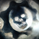จำนวนเข้าชม 2488
2488
RSI Breakout indicator, typically used on longer time frame (under Mid CAP) to find the next explosive stock. Can be used on monthly chart with 36 Months breakout period. Weekly with 52 Weeks Breakout period, 100 Days on daily, for Swing trading.
List of All my Indicators - https://www.tradingview.com/p/stocks/?so...
GL.
```study(title="UCS_Relative Strength Index With Breakout", shorttitle="UCS_RSI W/BO")

src = close
len1 = input(14, minval=1, title="Primary RSI Length")

up1 = rma(max(change(src), 0), len1)
down1 = rma(-min(change(src), 0), len1)

rsi1 = down1 == 0 ? 100 : up1 == 0 ? 0 : 100 - (100 / (1 + up1 / down1))

plot(rsi1, color=black)

length = input(60, minval=1, title="Breakout Length")
lower = lowest(rsi1,length)
upper = highest(rsi1,length)
basis = avg(upper, lower)

bo = rsi1 > upper
bd = rsi1 < lower

bcolor = bo ? green : bd ? red : na
plot(100, color=bcolor, style=circles, linewidth=4)
//plot(bo, color=green, title = 'Breakout', style = columns)
//plot(bd, color=red, title = 'Breakdown', style = columns)

h1=hline(70, "Overbought", red, solid, 3)
h2=hline(30, "Oversold", green, solid, 3)
h3=hline(50, "Median", black, dashed, 1)
fill(h1,h2, gray, 80, "Consolidation Zone")
```

## ไอเดียที่เกี่ยวข้องFirst Indicator is with 2 RSIs, so you measure the divergences. The second indicator, is with 1 RSI purely the breakout.
ตอบกลับ